# Class 9 Maths NCERT Solution for Heron Formula Ex 12.2

In this page we have NCERT book Solutions for Class 9th Maths:Heron Formula for EXERCISE 12.2 . Hope you like them and do not forget to like , social share and comment at the end of the page.

## Chapter 12 Ex 12.2

Question 1
A park, in the shape of a quadrilateral ABCD, has ∠C = 90º, AB = 9 m, BC = 12 m, CD = 5 m and AD = 8 m. How much area does it occupy?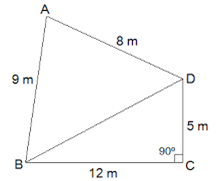Given in the question
∠C = 90º, AB = 9 m, BC = 12 m, CD = 5 m and AD = 8 m
BD is joined.
Area of the quadrilateral ABCD can be found using the area of the separate triangle and then adding up
In ΔBCD,
By applying Pythagoras theorem,
BD2 = BC2 + CD2
BD2 = 122 + 52
BD2 = 169
BD = 13 m

Area of ΔBCD=Area of right angle triangle= (1/2) × base × Height
So Area of ΔBCD = 1/2 × 12 × 5 = 30 m2
Now,
Semi perimeter of ΔABD(s) = (8 + 9 + 13)/2 m = 30/2 m = 15 m
Using heron's formula,
Area of ΔABD = √s (s-a) (s-b) (s-c)
= √15(15 - 13) (15 - 9) (15 - 8) m2
= √15 × 2 × 6 × 7 m2
= 6√35 m2 = 35.5 m2 (approx)

Area of quadrilateral ABCD = Area of ΔBCD + Area of ΔABD = 30 m2+ 35.5m2 = 65.5m2

Question 2
Find the area of a quadrilateral ABCD in which AB = 3 cm, BC = 4 cm, CD = 4 cm, DA = 5 cm and AC = 5 cm.
Given in the question
AB = 3 cm, BC = 4 cm, CD = 4 cm, DA = 5 cm and AC = 5 cm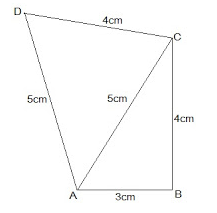Area of the quadrilateral ABCD can be found using the area of the separate triangle and then adding up
In ΔABC,
By applying Pythagoras theorem,
AC2 = AB2 + BC2
52 = 32 + 42
25 = 25
Thus, ΔABC is a right angled at B.

Area of ΔABC=Area of right angle triangle= (1/2) × base × Height
Area of ΔABC = 1/2 × 3 × 4 = 6 cm2
Now,
Semi perimeter of ΔACD(s) = (5 + 5 + 4)/2 cm = 14/2 cm = 7 m
Using heron's formula,
Area of ΔABD = √s (s-a) (s-b) (s-c)
= √7(7 - 5) (7 - 5) (7 - 4) cm2
= √7 × 2 × 2 × 3 cm2
= 2√21 cm2 = 9.17 cm2

Now Area of quadrilateral ABCD = Area of ΔABC + Area of ΔABD = 6 cm2+ 9.17 cm2 = 15.17 cm2

Question 3
Radha made a picture of an aeroplane with coloured paper as shown in below figure. Find the total area of the paper used.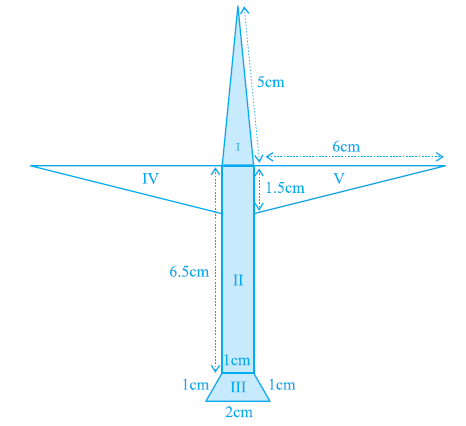Area of the aeroplane can be find using the area of the individual triangles and then summing them to get the total area
Area of triangle I

Length of the sides of the triangle section I = 5cm, 1cm and 5cm
Perimeter of the triangle = 5 + 5 + 1 = 11cm
Semi perimeter = 11/2 cm = 5.5cm
Using heron's formula,
Area of section I = √s (s-a) (s-b) (s-c)
= √5.5(5.5 - 5) (5.5 - 5) (5.5 - 1) cm2
= √5.5 × 0.5 × 0.5 × 4.5 cm2
= 0.75√11 cm2 = 0.75 × 3.317cm2 = 2.488cm2
Area of the rectangle II
Length of the sides of the rectangle of section II = 6.5cm and 1cm
Area of section II = 6.5 × 1 cm2 = 6.5 cm2
Area of the trapezium III
Section III is an isosceles trapezium which is divided into 3 equilateral of side 1cm each.
Area of the trapezium = 3 × √3/4 × 12cm2= 1.3 cm2
Area of triangle IV
Section IV is right angled triangles with base 6cm and height 1.5cm
Area of region IV = 1/2 × 6 × 1.5cm2= 4.5cm2
Area o f triangle V
Section IV is right angled triangles with base 6cm and height 1.5cm
Area of region IV = 1/2 × 6 × 1.5cm2= 4.5cm2

So Total area of the paper used = (2.488 + 6.5 + 1.3 + 4.5 + 4.5)cm2= 19.3 cm2

Question 4
A triangle and a parallelogram have the same base and the same area. If the sides of the triangle are 26 cm, 28 cm and 30 cm, and the parallelogram stands on the base 28 cm, find the height of the parallelogram.
Given,
Area of the parallelogram and triangle are equal.
Length of the sides of the triangle are 26 cm, 28 cm and 30 cm.
Perimeter of the triangle = 26+ 28 + 30 = 84 cm
Semi perimeter of the triangle = 84/2 cm = 42 cm
Using heron's formula,
Area of the triangle = √s (s-a) (s-b) (s-c)
= √42(42 - 26) (46 - 28) (46 - 30) cm2
= √46 × 16 × 14 × 16 cm2
= 336 cm2Let height of parallelogram be h.
Now given
Area of parallelogram = Area of triangle
Base × h = 336 cm2
28cm × h = 336 cm2
h = 336/28 cm
h = 12 cm
The height of the parallelogram is 12 cm.

Question 5
A rhombus shaped field has green grass for 18 cows to graze. If each side of the rhombus is 30 m and its longer diagonal is 48 m, how much area of grass field will each cow be getting?
let PQRS be the rhombus shaped field and Diagonal QS divides the rhombus PQRS into two congruent triangles of equal area.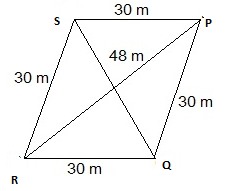Semi perimeter of ΔPQS = (30 + 30 + 48)/2 m = 54 m
Using heron's formula,
Area of the ΔPQS = √s (s-a) (s-b) (s-c)
= √54(54 - 30) (54 - 30) (54 - 48) m2
= √54 × 14 × 14 × 6 cm2
= 432 m2
Area of field = 2 × area of the ΔPQS = (2 × 432)m2= 864 m2
So,
Area of grass field which each cow will be getting = 864/18 m2=48 m2

Question 6
An umbrella is made by stitching 10 triangular pieces of cloth of two different colors as shown below in figure. Each piece measuring 20 cm, 50 cm and 50 cm. How much cloth of each colour is required for the umbrella?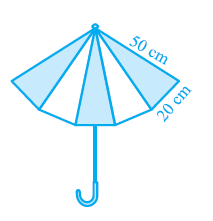The total Umbrella area is equal 5 times area of one triangle.
Semi perimeter of each triangular piece = (50 + 50 + 20)/2 cm = 120/2 cm = 60cm
Using heron's formula,
Area of the triangular piece = √s (s-a) (s-b) (s-c)
= √60(60 - 50) (60 - 50) (60 - 20) cm2
= √60 × 10 × 10 × 40 cm2
= 200√6 cm2
Area of triangular piece = 5 × 200√6 cm2 = 1000√6 cm2

Question 7
A kite in the shape of a square with a diagonal 32 cm and an isosceles triangle of base 8 cm and sides 6 cm each is to be made of three different shades as shown in below. How much paper of each shade has been used in it?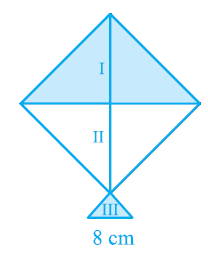We know from the property of square the diagonals of a square bisect each other at right angle.
Also Area of given kite = 1/2 (diagonal)2
= 1/2 × 32 × 32 = 512 cm2
So it is equal to
= 512/2 cm2= 256 cm2
So, area of paper required in each shade = 256 cm2
For the III section, It is simple area calculation
Length of the sides of triangle = 6cm, 6cm and 8cm
Semi perimeter of triangle = (6 + 6 + 8)/2 cm = 10cm
Using heron's formula,
Area of the III triangular piece = √s (s-a) (s-b) (s-c)
= √10(10 - 6) (10 - 6) (10 - 8) cm2
= √10 × 4 × 4 × 2 cm2
= 8√6 cm2

Question 8
A floral design on a floor is made up of 16 tiles which are triangular, the sides of the triangle being 9 cm, 28 cm and 35 cm . Find the cost of polishing the tiles at the rate of 50p per cm2 .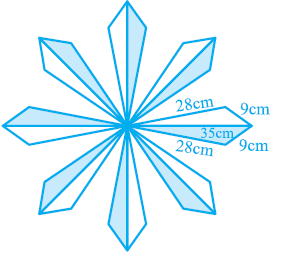Semi perimeter of each triangular shape = (28 + 9 + 35)/2 cm = 36 cm
Using heron's formula,
Area of each triangular shape = √s (s-a) (s-b) (s-c)
= √36(36 - 28) (36 - 9) (36 - 35) cm2
= √36 × 8 × 27 × 1 cm2
= 36√6 cm2= 88.2 cm2
Total area of 16 tiles = 16 × 88.2 cm2 = 1411.2 cm2Cost of polishing tiles = 50p per cm2
Total cost of polishing the tiles = Rs. (1411.2 × 0.5) = Rs. 705.6

Question 9
A field is in the shape of a trapezium whose parallel sides are 25 m and 10 m. The non-parallel sides are 14 m and 13 m. Find the area of the field.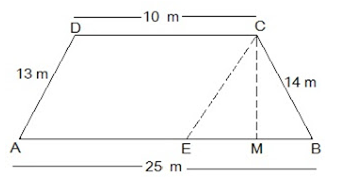Let ABCD be the given trapezium with parallel sides AB = 25m and CD = 10m and the non-parallel sides AD = 13m and BC = 14m.
We can drop a perpendicular from C on AM and draw a line CE parallel to DA
CM ⊥ AB and CE || AD.
This will divide the trapezium into two part
(i) Parallelogram
(ii) Triangle
Now
In ΔBCE,
BC = 14m, CE = AD = 13 m and
BE = AB - AE = 25 - 10 = 15m
Semi perimeter of the ΔBCE = (15 + 13 + 14)/2 m = 21 m
Using heron's formula,
Area of the ΔBCE = √s (s-a) (s-b) (s-c)
= √21(21 - 14) (21 - 13) (21 - 15) m2
= √21 × 7 × 8 × 6 m2
= 84 m2
Now area of the ΔBCE = 1/2 × BE × CM = 84 m2
1/2 × 15 × CM = 84 m2
CM = 168/15 m2
CM = 56/5 m2
Area of the parallelogram AECD = Base × Altitude = AE × CM = 10 × 84/5 = 112 m2
Area of the trapezium ABCD = Area of AECD + Area of ΔBCE
= (112+ 84) m2 = 196 m2

## Summary

1. NCERT solutions for class 9 maths chapter 12 Heron Formula Ex 12.2 has been prepared by Expert with utmost care. If you find any mistake.Please do provide feedback on mail. You can download the solutions as PDF in the below Link also
2. This chapter 12 has total 2 Exercise 12.1 and 12.2. This is the second exercise in the chapter.